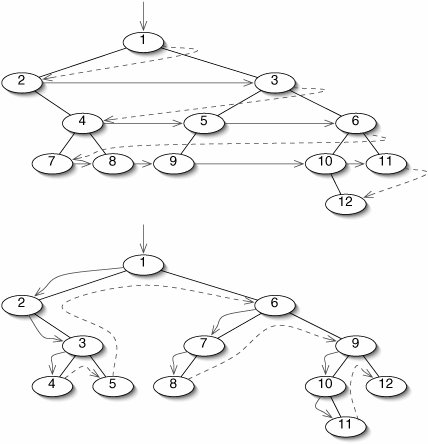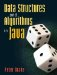# Tree Traversal

In Section 5.4, we discussed traversing linear structuresthat is, visiting each item in turn. We have traversed linear structures using Iterators and in methods such as toString().

A linear structure is normally traversed from front to back. Occasionally it is useful to traverse one from back to front. With trees, the order of traversal is less obvious. The root should probably be either first or last, but what about the other nodes?

It turns out that there are four meaningful orders in which to traverse a binary tree: preorder, inorder, postorder, and level order. For reference, the four traversals of the tree in Figure 10-4 are shown in Figure 10-14.

Figure 10-14. Traversing the binary tree in Figure 10-4.

Traversal Order

Order in which Nodes are Visited

Preorder

AGEKLHBFICJD

Inorder

GKELAFBHCJID

Postorder

KLEGFBJCDIHA

Level order

AGHEBIKLFCDJ

The first three orders have very elegant algorithms based on the recursive structure of a tree. Indeed, these algorithms are generally given as definitions of the traversal orders. For example, the algorithm for preorder traversal is:

1. Visit the root.
2. Recursively traverse the left subtree preorder.
3. Recursively traverse the right subtree preorder.

This algorithm is easily translated into a method for the BinaryNode class (Figure 10-15). Note that the base case is handled in an unusual way here. Rather than checking for the base case (an empty tree) at the beginning of the method, we check before each recursive invocation. This is necessary because the empty tree is represented by null, and we can't invoke methods on null.

Figure 10-15. This method for the BinaryNode class traverses a tree preorder.

 ``` 1 /** 2 * Return a String representation of the tree rooted at this node, 3 * traversed preorder. 4 */ 5 public String toStringPreorder() { 6 String result = ""; 7 result += item; 8 if (left != null) { 9 result += left.toStringPreorder(); 10 } 11 if (right != null) { 12 result += right.toStringPreorder(); 13 } 14 return result; 15 } ```

The inorder traversal algorithm is almost identical, except that we traverse the left subtree before visiting the root (Figure 10-16).

Figure 10-16. The only difference between preorder traversal (Figure 10-15) and inorder traversal is the order of lines 713.

 ``` 1 /** 2 * Return a String representation of the tree rooted at this node, 3 * traversed inorder. 4 */ 5 public String toStringInorder() { 6 String result = ""; 7 if (left != null) { 8 result += left.toStringInorder(); 9 } ``` 10 result += item; 11 if (right != null) { 12 result += right.toStringInorder(); 13 } 14 return result; 15 }

Visiting the root after both subtrees results in a postorder traversal (Figure 10-17).

Figure 10-17. In a postorder traversal, the root is visited after both subtrees.

 ``` 1 /** 2 * Return a String representation of the tree rooted at this node, 3 * traversed postorder. 4 */ 5 public String toStringPostorder() { 6 String result = ""; 7 if (left != null) { 8 result += left.toStringPostorder(); 9 } 10 if (right != null) { 11 result += right.toStringPostorder(); 12 } 13 result += item; 14 return result; 15 } ```

As we mentioned in Section 9.5, any recursive algorithm can be converted into an iterative one by explicitly simulating the call stack. While this is generally not worth the effort, it is instructive to look at an iterative version of toStringPreorder(). It turns out that, for this particular algorithm, we don't have to store complete call frames on the stackjust the root of each subtree to be traversed (Figure 10-18).

Figure 10-18. An iterative version of toStringPreorder() using an explicit stack. It is necessary to push the right child before the left child because of the last-in, first-out policy of a stack.

 1 /** 2 * Return a String representation of the tree rooted at this node, 3 * traversed preorder. 4 */ 5 public String toStringPreorder() { 6 String result = ""; 7 Stack> stack = new ArrayStack>(); 8 stack.push(this); 9 while (!(stack.isEmpty())) { 10 BinaryNode node = stack.pop(); 11 result += node.item; 12 if (node.right != null) { 13 stack.push(node.right); 14 } 15 if (node.left != null) { 16 stack.push(node.left); 17 } 18 } 19 return result; 20 }

The stack holds the roots of the subtrees we have yet to traverse. In the example of Figure 10-4, while we are traversing the subtree rooted at G, G's sibling H is at the bottom of the stack, waiting its turn. As we find proper descendants of G, they are pushed onto the top of the stack, so they are handled before H.

A curious thing happens if we replace the stack with a queue. Now G's proper descendants are handled after H. If we add the left child to the queue before the right child, we get the algorithm for the level order traversal (Figure 10-19). The root is visited first, then all of the nodes at depth 1, then depth 2, and so on.

Figure 10-19. Changing the stack to a queue and swapping the order of child insertion results in the algorithm for level order traversal.

 ``` 1 /** 2 * Return a String representation of the tree rooted at this node, 3 * traversed level order. 4 */ 5 public String toStringLevelOrder() { 6 String result = ""; 7 Queue> q = new ArrayQueue>(); 8 q.add (this); 9 while (!(q.isEmpty())) { 10 BinaryNode node = q.remove (); 11 result += node.item; 12 if (node.left != null) { 13 q.add(node.left); 14 } 15 if (node.right != null) { 16 q.add(node.right); 17 } 18 } 19 return result; 20 } ```

Level order traversal is sometimes called breadth-first because it traverses all the way across each level before going deeper (Figure 10-20). The other traversals are called depth-first because they go all the way down to a leaf before "backing up" and trying a different path.

Figure 10-20. A breadth-first traversal (top) visits every node in each level before going on to the next level. A depth-first traversal (bottom) goes all the way down to a leaf before "backing up" and traversing a different subtree.Any traversal takes time in Q(n) because it does a constant amount of work visiting each node. If the order isn't important and the tree is perfect or close to perfect, depth-first traversals are more efficient. The issue is not time but space. A depth-first traversal uses space for the call stack. The number of call frames is proportional to the height of the tree, which is in Q(log n) in a perfect tree. A breadth-first traversal, on the other hand, uses space for a queue. This can be as large as an entire level of the tree, which is in Q(n) in a perfect tree.

Exercises

 10.6 In the preorder tree traversal, the base case is an empty tree. Would a leaf be a legitimate base case? Explain. 10.7 Is the order in which nodes are visited in a postorder traversal the reverse of the order produced by a preorder traversal? Explain. 10.8 Does a depth-first or a breadth-first traversal use more memory when traversing a linear tree? 10.9 Project 4.20 defined infix and postfix notation. Draw a binary tree which produces the infix expression 3 + 2 * 4 when traversed inorder and the postfix expression 3 2 4 * + when traversed postorder. What expression is produced if this tree is traversed preorder?Data Structures and Algorithms in Java
ISBN: 0131469142
EAN: 2147483647
Year: 2004
Pages: 216
Authors: Peter Drake